# Normalization Scheme

The Alfvén speed, which is the typical phase velocity of a compressible-Alfvén wave, is defined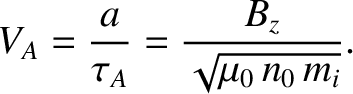(4.23)

[See Equation (3.181).] It is helpful to define the collisionless ion skin-depth,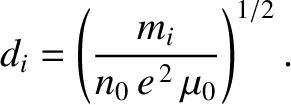(4.24)

Note that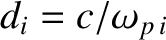, where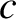is the velocity of light in vacuum, and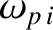the ion plasma frequency .

Let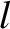be a typical variation lengthscale in the resonant layer. It is convenient to adopt the following normalization scheme that renders all quantities in the drift-MHD fluid equations dimensionless: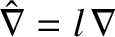,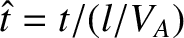,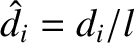,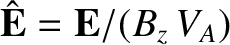,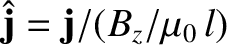,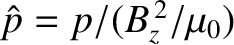,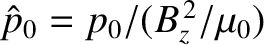,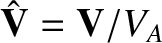,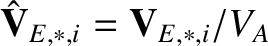,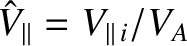,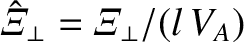,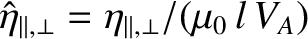, and. Equations (4.7)–(4.13) and (4.20)–(4.22) yield the following set of normalized drift-MHD fluid equations: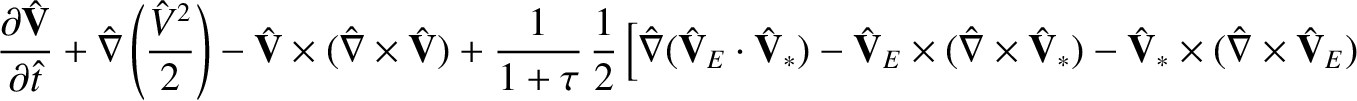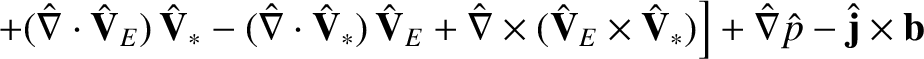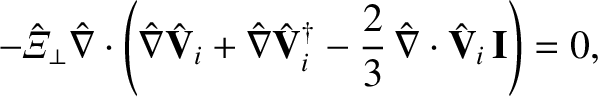(4.25)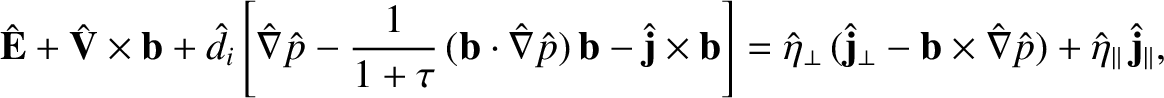(4.26)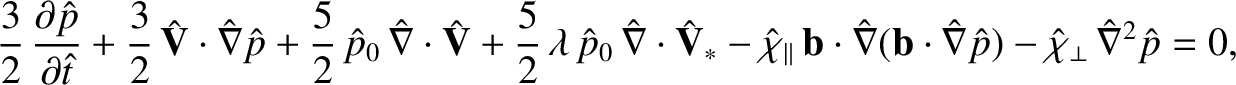(4.27)

where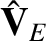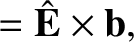(4.28)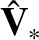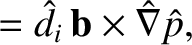(4.29)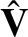(4.30)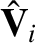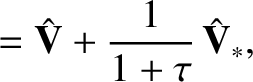(4.31)

and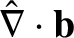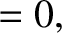(4.32)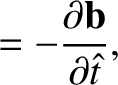(4.33)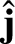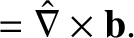(4.34)

Here, use has been made of some standard vector identities.